×

Search anything:

# Multithreaded Matrix Multiplication in C++

#### C++ Deep LearningGet this book -> Problems on Array: For Interviews and Competitive Programming

In this article, we will explored how to implement Multithreaded Matrix Multiplication in C++ Programming Language. Matrix Multiplication is a critical operation in Deep Learning and this makes this topic critical.

Matrix multiplication is a binary operation that produces a matrix from two matrices. For this operation the only one condition is the number of columns of the first matrix must be equal to the number of rows of the second matrix. If there are two matrices, `A` and `B` then their product is denoted by `AB`. So if the operation produces a matrix `C` then we can write it as `AB = C`. Here `C` is also known as matrix product. When we talk about normal multiplication, its commutative, which means if `a` and `b` are two numbers then `a * b = b * a`, but in case of matrices those wont be equal and most of the time the operation would be impossible to execute as it will not meet the condition to execute the process.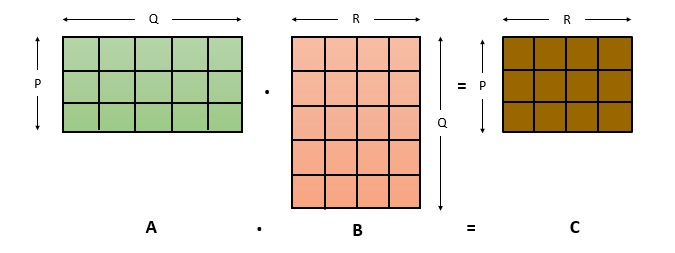Since we got to know about the operation. Lets move on to the contents. To see the code which I will be referring here, check out this repository, it is recommended to use linux based OS to run the program.

• Approach
• Implementation Details
• Working
• Output
• Conclusion

## Approach

So here we will be using threads to help us calculate the matrix product. In normal approach we use nested loops to multiply the two matrix. This process would involve using nested loops to iterate through the rows and columns of the matrices. This is the normal approach and it results in O(N^3) time complexity, where N is the size of the matrix. So this could take a long time if we are dealing with a big matrix, and if this is used in a application it can also effect the performance of the application as a whole.

Here we will be using the multi-thread approach which will distribute the work among multiple threads, which significantly reduced the time required to complete the multiplication and will also improve the performance of the application.
Here with this process we can utilize the processing power of multiple cores, and each thread can work on a small separate potion of the matrices simultaneously. This will help us to maximize the use of system resources and reduce the overall execution time of the program.

Now lets look at how this operation is done. So first we will make sure that the given matrices follow the condition, which is the number of columns of the first matrix must be equal to the number of rows of the second matrix. Let A be an m x n matrix and B be an n x p matrix. The resulting matrix C is an m x p matrix, where the element in the ith row and jth column is obtained by taking the dot product of the ith row of A and the jth column of B:

``````C[i][j] = A[i]*B[j] + A[i]*B[j] + ... + A[i][n]*B[n][j]
``````

Lets understand this in a fun way, check the image below.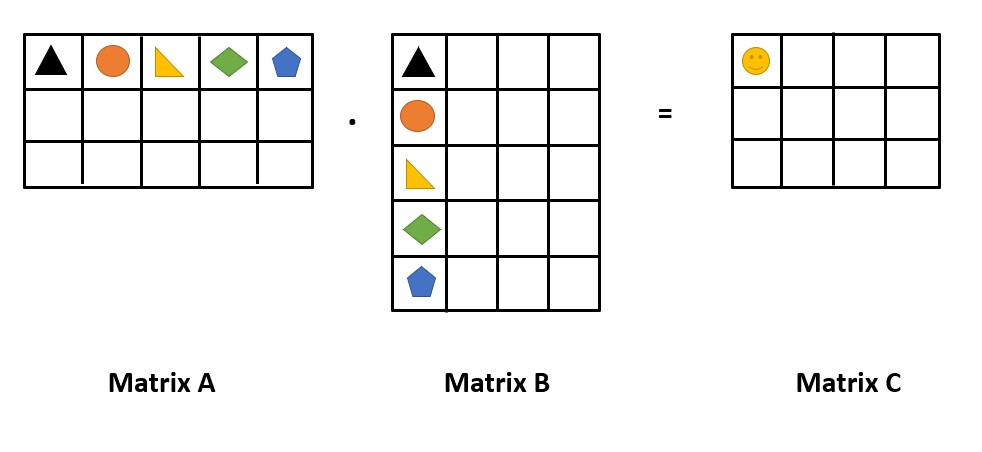In the picture we have two matrices A & B which are both multiplied to to get a matrix C. So here we can see that to get the value inside the 😀 cell we have to multiply the similar shapes in the two matrices and add them.

So here `😀 = (triangle*triangle)+(circle*circle)+...+(pentagon*pentagon)`

The pseudo code for this operation is:

``````1. Accept the matrices and verify whether it follows the condition.
2. Create a new matrix C with rows same as that of A and columns same as that of B
3. For each row i in A:
a. For each column j in B:
i. For each element k in the row i of A and the column j of B:
a. Add the product of A[i][k] and B[k][j] to C[i][j].
``````

So which part is being handled by the threads. The operations handled by the threads run parallelly, so lets check out what does it handle.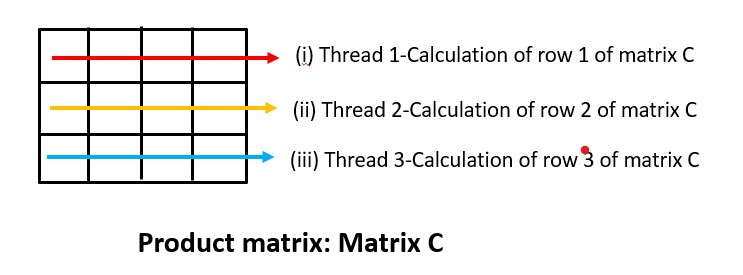So the calculation of `(i), (ii) and (iii)` are handled by the threads and these calculations run parallelly. The calculation of the four cells present in the row `1` of the matrix C is being handled by the thread `1` and the row `2` is being handled by the thread `2` and the row `3` by the thread `3`.

## Implementation Details

• Threads: Threads are a way to achieve concurrent execution in a program, by allowing multiple parts of the program to execute independently and simultaneously. Here in C++ threads are executed using threads class which is a part of the STL.
Threads share same memory space and resources with the parent process, but operate in their own stack and program counter which allows them to execute independently.

• `pthread_t`: It is a data type in C++ that represents a thread. It is defined in `pthread.h` header file and is used to create, manage and synchronize threads in a multi-thread application. Threads are implemented as lightweight processes that share the same address space and resources of the parent process. `pthread_t` provides a unique identifier for each thread.

• `pthread_create()`: It is a function used to create new thread. It takes in four arguments:

• The first one is a pointer to a `pthread_t` variable, which would get set to the thread ID of the new thread.
• The second one is a pointer to a structure, which specifies the attributes of the new thread. We will be passing `NULL` and in that case default attributes are used.
• Third will be a pointer to the function that will be run by the new thread.
• The last one is an optional.
• `pthread_join()`: It is a function in C++ that is used to wait for a thread to terminate before the program continues execution. It is also defined in the `pthread.h` header file and takes in two arguments, which are a `pthread_t` variable that represents the thread to join and a pointer to a location where the exit status of the thread will be stored. Generally the main thread finishes before the child thread could finish, but when `pthread_join` is called, the program wait until the specified thread finishes executing, and then main thread will continue its execution.

• `void* arg`: The `void* arg` parameter is a pointer to the argument passed to the function when it is called by `pthread_create`.

• `void* multi`: These types of functions have `void*` return type, which means it does not return a value. The `void*` returns a generic pointer that can be cast to the appropriate type by the calling thread.

• `rand()`: It is a built-in function in C++ that generates a pseudo-random number. The function returns an integer value between 0 and RAND_MAX (which is a constant defined in the `<cstdlib>` header file).

## Working

In the beginning we are declaring some global variables `p`,`q`,`r` these are the number of rows and columns of the two matrices. Here we also declare the `Number_of_Threads` variable, so that all these variables can be accessed from anywhere in the program.

``````int Number_of_Threads;
int p, q, r;
``````

First in the program after we display the title we give the warning that the number of threads is equal to the `p` value. This is because each thread would be given the task to find out the first row of the product matrix and the number of rows for the product matrix is `p`. So enter values according to the resource available in your system.

``````cout << "                \033[33mMatrix Multiplication Using Threads\033[0m" << endl
<< "-------------------------------------------------------------------" << endl
<< "        \033[31mNote: The thread number would be given the value of p " << endl
<< "         so enter the value according to available resources.\033[0m" << endl
<< "Rows and columns of matrix A are p & q" << endl
<< "Rows and columns of matrix B are q & r" << endl
<< endl
<< "Enter number of rows (p) & number of columns (q) of matrix A: ";
cin >> p >> q;
cout << "Enter number of columns (r) of matrix B: ";
cin >> r;
``````

So in the above code we accepts the values for `p`, `q` and `r` and `Number_of_Threads = p`. Now we need to give the matrices its size, so for that we use `resize()` function, which would resize the vector, and then we use a for loop to resize the rows of the matrix. We can also use`matA.assign(p, vector<int>(q, 0));` expression to initialize the size and give all the values as zero.

``````for (int i = 0; i < p; i++)
{
matrixA[i].resize(q);
}
matrixB.resize(q);
for (int i = 0; i < q; i++)
{
matrixB[i].resize(r);
}
matrixC.resize(p);
for (int i = 0; i < p; i++)
{
matrixC[i].resize(r);
}
``````

Now we give a choice to the user, whether they want to enter the values or just want to see the demonstration. If they don't want to enter values, random values are given to the matrices using the `rand()` function, else the values are accepted from the user.
This is handled using a switch case.

``````switch (choice)
{
case 'y':
case 'Y':
cout << "Enter the values for matrix A: ";
for (int i = 0; i < p; i++)
{
for (int j = 0; j < q; j++)
{
cin >> matrixA[i][j];
}
}
cout << "Enter the values for matrix B: ";
for (int i = 0; i < q; i++)
{
for (int j = 0; j < r; j++)
{
cin >> matrixB[i][j];
}
}
break;

default:
for (int i = 0; i < p; i++)
{
for (int j = 0; j < q; j++)
{
matrixA[i][j] = rand() % 10;
}
}
for (int i = 0; i < q; i++)
{
for (int j = 0; j < r; j++)
{
matrixB[i][j] = rand() % 10;
}
}
break;
}
``````

After this we will be having our both matrices, now before calculating we will first display both matrices in order.

`````` for (int i = 0; i < p; i++)
{
for (int j = 0; j < q; j++)
cout << matrixA[i][j] << " ";
cout << endl;
}

cout << endl
<< "Matrix B:" << endl;
for (int i = 0; i < q; i++)
{
for (int j = 0; j < r; j++)
cout << matrixB[i][j] << " ";
cout << endl;
}
``````

Now we will get onto the calculation part. Here first we will declare `pthread_t` data type variable, to initialize the threads. This step will create the number of threads we need. As we said before this value would be equal to `p`. Now we would use a `for` loop and create all the threads using `pthread_create()` and we would pass in all the values, along with the function the thread has to perform, here it is the `operation()` function.

``````    pthread_t threads[Number_of_Threads];

for (int i = 0; i < Number_of_Threads; i++)
{
int *point;
}
``````

Now lets look at the `operation()` function. This function returns a pointer to the function and it also accepts a pointer argument. We would declare a variable called `rowOfC` which indicates, which row the function is evaluating, and after the `for` loops we will increment the value by one so that we can compute the next row. Here in this function we are returning `NULL` as we don't have a pointer variable to return and we are only using a pointer function as the `pthread_join()` expects one. So using the `i` and the two `for` loops we compute one row of the product matrix and this function will be executed by `p` number of threads which is equal to `Number_of_Threads` variable.

``````void *operation(void *arg)
{
int i = rowOfC;

for (int j = 0; j < r; j++)
for (int k = 0; k < q; k++)
matrixC[i][j] += matrixA[i][k] * matrixB[k][j];
++rowOfC;
return NULL;
}
``````

After this step we will use `pthread_join()` which will wait for each of the threads to finish and then continues the program.

``````for (int i = 0; i < Number_of_Threads; i++)
``````

Now we will have the product matrix stored in `matrixC`, and we will display it using the for loops, and the program ends.

``````for (int i = 0; i < p; i++)
{
for (int j = 0; j < r; j++)
cout << matrixC[i][j] << " ";
cout << endl;
}
``````

## Output

When matrix is generated randomly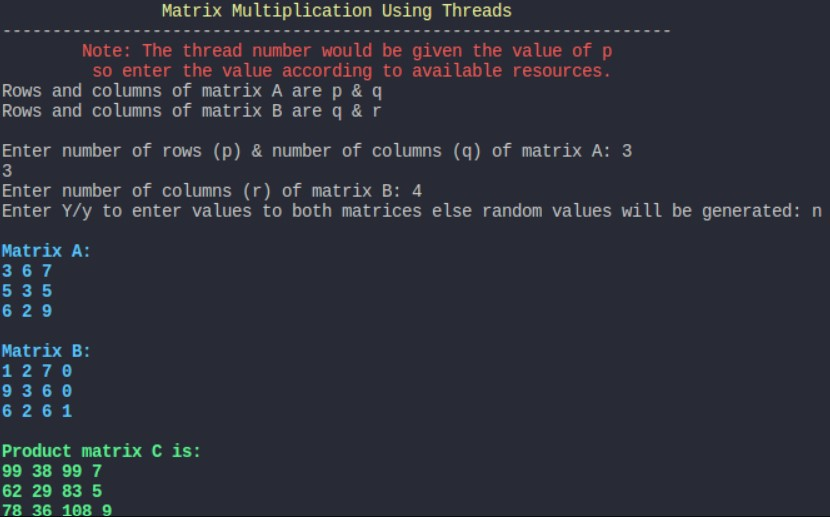When user inputs the matrix values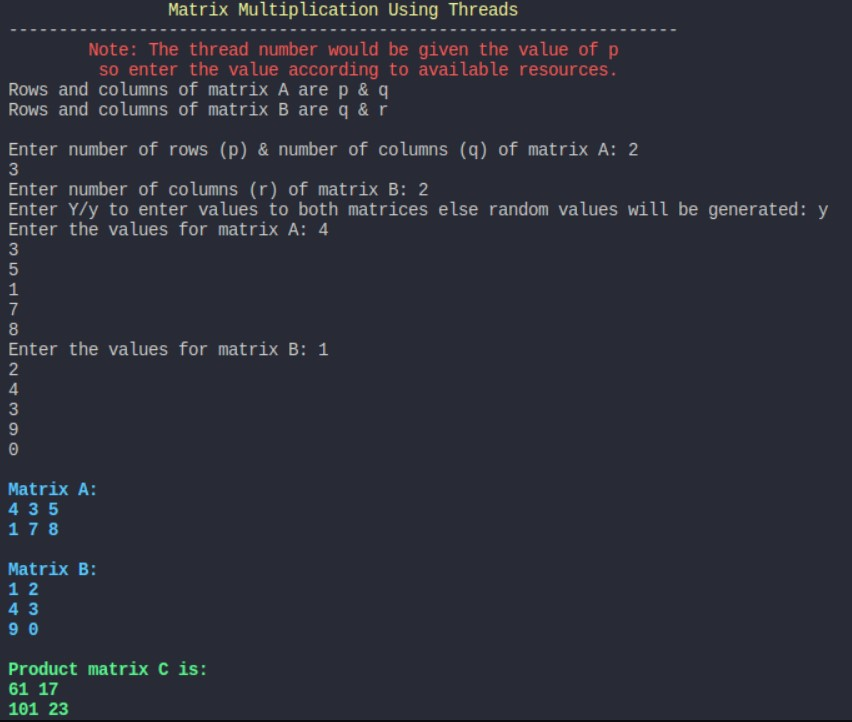## Conclusion

So by the end of this article you will have a complete idea on how this program works, and why we do it.

In the starting part we discussed the normal time complexity which was `N^3`, so lets check how has it improved. So if we use threads we can reduce the time complexity from `N^3` to `(N^3)/P` where `N` is the dimension of the matrix and `P` is the number of threads running parallelly. The division by `P` indicates that the work is divided among `P` threads, each responsible for computing a portion of the final product matrix.

Matrix multiplication is one of the fundamental operation in mathematics and computer science which has a wide range of applications in various fields. Some of it includes graphics, image processing, signal processing, machine learning, cryptography, physics etc.

Parallelization of matrix multiplication using threads is a common optimization technique for speeding up the computation of large matrices. This is because matrix multiplication involves a large number of calculations, and dividing the calculation into multiple threads can help to leverage the multi-core processing power of modern computers. Still there are other techniques for parallelizing matrix multiplication, like using GPUs, hardware accelerators etc. The choice of the technique will depend on the size of the matrix and the resources available and application requirements.

#### Aswin Shailajan

Aswin Shailajan is a B. Tech Computer Science student at Vellore Institute of Technology and is a SDE, Intern at OpenGenus.